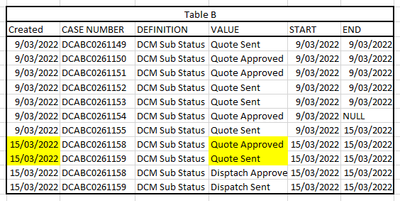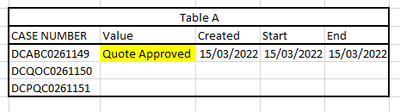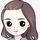cancel
Showing results for
Did you mean:Helper I

## Using dax to get latest value from another table

I'd like to update table A with values from Table B.
Requirement - to update the latest [Value] based on [Created] where [value] contains 'Quote'.
Catch is, if on the same date quote is sent and approved, then table A needs to be updated with Quote Approved. What is the best way to get the desired outcome.

``````Quote_Sub =
VAR CaseNumber = 'A'[CASE NUMBER]
VAR SUB_Table =
CALCULATETABLE (
SELECTCOLUMNS (
FILTER ( B, B[Case Number] = CaseNumber ),
"Start Date", B[Created],
"Sub Value", B[Value]
),
CONTAINSSTRING ( DCM_METRIC_INSTANCE[Value], "Quote" )
)
VAR Quote_A = MAXX(FILTER(SUB_Table, [Sub Value] = "Quote Approved"),[Start Date])
VAR Quote_Ex = MAXX(FILTER(SUB_Table, [Sub Value] = "Quote Expired"),[Start Date])
VAR Quote_R = MAXX(FILTER(SUB_Table, [Sub Value] = "Quote Rejected"),[Start Date])
VAR Quote_Es = MAXX(FILTER(SUB_Table, [Sub Value] = "Quote Escalated"),[Start Date])
VAR Quote_S = MAXX(FILTER(SUB_Table, [Sub Value] = "Quote Sent"),[Start Date])

VAR max_date = MAXX({Quote_A,Quote_Ex,Quote_R,Quote_Es,Quote_S}, [Value])
VAR max_value =
calculatetable(
FIRSTNONBLANK(SELECTCOLUMNS( FILTER(SUB_Table,[Start Date]=max_date),
"max Value",[Sub Value]),[max Value])
)
return max_value``````1 ACCEPTED SOLUTIONCommunity Support

Hi @lalTel20 ,

You can create a calculated column as below in table A, please find the details in the attachment.

 Quote_Sub = VAR _acnum = 'A'[CASE NUMBER]VAR _maxdate =CALCULATE ( MAX ( 'B'[Created] ), FILTER ( 'B', 'B'[CASE NUMBER] = _acnum ) )VAR _count =CALCULATE (DISTINCTCOUNT ( 'B'[VALUE] ),FILTER ('B','B'[CASE NUMBER] = _acnum&& 'B'[VALUE]IN { "Quote Approved", "Quote Sent" }&& 'B'[Created] = _maxdate))RETURNIF ( _count = 2, "Quote Approved", 'A'[Value] )If the above one is not your expected one, please provide some sample data (exclude sensitive data) in the table A and B with Text format and your expected resultwith backend logic and special examples. It is better if you can share a simplified pbix file with me. You can refer the following thread to upload your file in the community. Thank you.

How to upload PBI in Community

Best Regards

Community Support Team _ Rena
If this post helps, then please consider Accept it as the solution to help the other members find it more quickly.
3 REPLIES 3Community Support

Hi @lalTel20 ,

You can create a calculated column as below in table A, please find the details in the attachment.

 Quote_Sub = VAR _acnum = 'A'[CASE NUMBER]VAR _maxdate =CALCULATE ( MAX ( 'B'[Created] ), FILTER ( 'B', 'B'[CASE NUMBER] = _acnum ) )VAR _count =CALCULATE (DISTINCTCOUNT ( 'B'[VALUE] ),FILTER ('B','B'[CASE NUMBER] = _acnum&& 'B'[VALUE]IN { "Quote Approved", "Quote Sent" }&& 'B'[Created] = _maxdate))RETURNIF ( _count = 2, "Quote Approved", 'A'[Value] )If the above one is not your expected one, please provide some sample data (exclude sensitive data) in the table A and B with Text format and your expected resultwith backend logic and special examples. It is better if you can share a simplified pbix file with me. You can refer the following thread to upload your file in the community. Thank you.

How to upload PBI in Community

Best Regards

Community Support Team _ Rena
If this post helps, then please consider Accept it as the solution to help the other members find it more quickly.Super User

@lalTel20 , Is there a preference when date is the same

example measure

new column =
var _max = maxx(filter(Table, [casenumber] = earlier([casenumber]) ), [Created])
return
maxx(filter(Table, [casenumber] = earlier([casenumber]) && [Created] = _max), [Value])Helper I

Hi @amitchandak
Thank you for your response. Yes the preference is Quote sent gets the least preference, since after the quote is sent, it can be rejected, accepted, escalated or expired on the same day. And it is not always necessary that there will be a subsequent event for a casenumber. In such scenario the atest stage would be quote sent.Announcements#### Exclusive opportunity for Women!

Join us for a free, hands-on Microsoft workshop led by women trainers for women where you will learn how to build a Dashboard in a Day!#### Power Platform Conference-Power BI and Fabric Sessions

Join us Oct 1 - 6 in Las Vegas for the Microsoft Power Platform Conference.Top Solution Authors
Top Kudoed Authors
Users online (1,537)